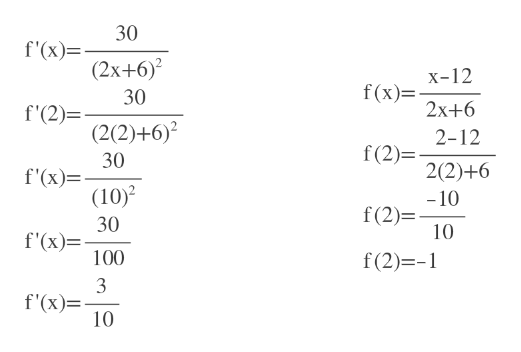# Let f(x) = (x - 12) / (2x + 6)Find an equation for the tangent line to the graph of f at x = 2y = ?

Question
1 views

Let f(x) = (x - 12) / (2x + 6)

Find an equation for the tangent line to the graph of f at x = 2

y = ?

check_circle

Step 1

We find f'(x) first using quotient rule

Step 2

Slope= f'(2)

And y=f(2)

So, slope=...help_outlineImage Transcriptionclose30 f'(x)= (2x+6)2 x-12 f(x)= 30 2x+6 f'(2)= (2(2)+6) 2-12 f(2) 30 f'(x)= (10)2 2(2)+6 -10 f(2) 10 30 f'(x) 100 f(2) - f'(x) 10 fullscreen

### Want to see the full answer?

See Solution

#### Want to see this answer and more?

Solutions are written by subject experts who are available 24/7. Questions are typically answered within 1 hour.*

See Solution
*Response times may vary by subject and question.
Tagged in

### Derivative